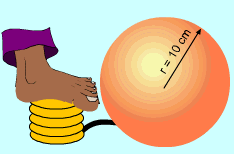Ch 3. Derivatives II Multimedia Engineering Math Higher OrderDerivatives RelatedRates Differential Newton'sMethod
 Chapter 1. Limits 2. Derivatives I 3. Derivatives II 4. Mean Value 5. Curve Sketching 6. Integrals 7. Inverse Functions 8. Integration Tech. 9. Integrate App. 10. Parametric Eqs. 11. Polar Coord. 12. Series Appendix Basic Math Units Search eBooks Dynamics Fluids Math Mechanics Statics Thermodynamics Author(s): Hengzhong Wen Chean Chin Ngo Meirong Huang Kurt Gramoll ©Kurt GramollMATHEMATICS - CASE STUDY IntroductionEnlarging Balloon In order to decorate a living room for a birthday party, Alice is pumping air into balloons. She is curious about the balloons' surface rate of change when the radius of the balloon is 10 cm. What is known: The rate of air being pumped into a balloon is 8 cm3/sec. The radius is 10 cm. Questions What is the surface rate of change of the balloon? Approach This problem needs to find the surface area changes with respect to time.